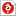검색어 입력폼

Motor Control

저작시기 2011.11 |등록일 2012.05.14한컴오피스 (hwp) | 14페이지 | 가격 1,000원

소개글

성대 진동실험 A+ 받은 레포트 입니다

목차

1. Principles of Feedback
2. Proportional Position Control
3. Position Control with Velocity Feedback

본문내용

-Preliminary report
1. Principles of Feedback
A. Introduction to Feedback Control
We have studied the characteristics of 2nd order linear system without control applied. In this section, we shall see the effect of adding feedback and perform experiments to control a DC motor.
A general control system is usually represented by a block-diagram as shown in Fig. 5.1. There is a variable whose value is to be controlled (output) and a variable which represents the value of the output required (reference input r). This output is measured by a sensor and compared to the reference and generates the error(e). In general, the sensor has its own dynamics, but it is assumed to be identity in this lab for the sake of simplicity. Therefore, we have and . The error is manipulated by the controller to provide the drive or control input to the plant (u) which is the signal that tells the plant what to do.
By measuring what the output is doing and feeding this back to be compared with the input, a Closed Loop Feedback Control System has been created.

참고 자료

진동 및 동적 시스템 실험 manual(English+version)
http://en.wikipedia.org/wiki/Noise_(electronics)
http://en.wikipedia.org/wiki/Rise_time